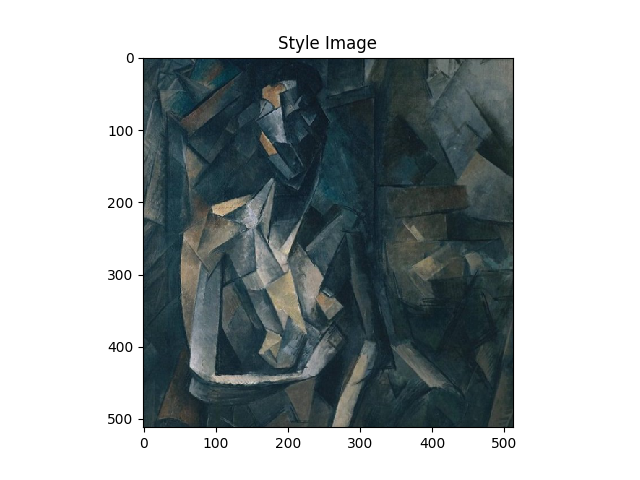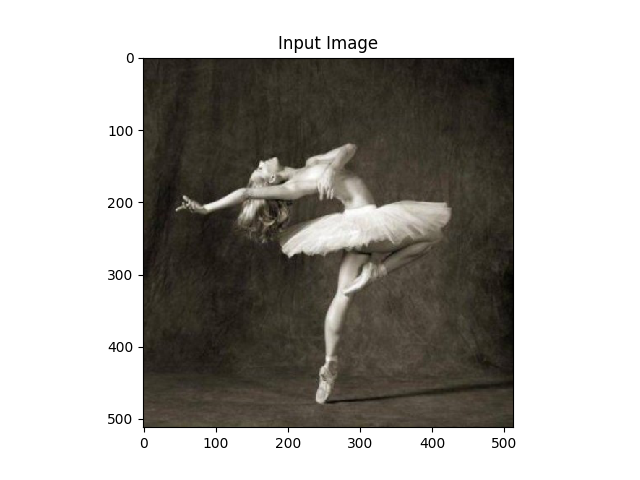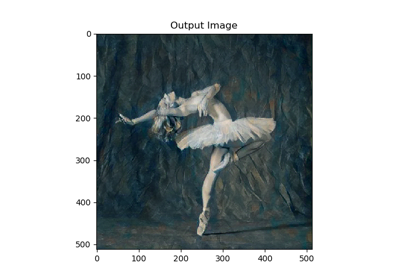# 使用 PyTorch 进行 Neural-Transfer

### 3.导包并选择设备

from __future__ import print_function

import torch
import torch.nn as nn
import torch.nn.functional as F
import torch.optim as optim

from PIL import Image
import matplotlib.pyplot as plt

import torchvision.transforms as transforms
import torchvision.models as models

import copy


device = torch.device("cuda" if torch.cuda.is_available() else "cpu")


### 4.加载图片

# 所需的输出图像大小
imsize = 512 if torch.cuda.is_available() else 128  # use small size if no gpu

transforms.Resize(imsize),  # scale imported image
transforms.ToTensor()])  # transform it into a torch tensor

image = Image.open(image_name)
# fake batch dimension required to fit network's input dimensions
return image.to(device, torch.float)

assert style_img.size() == content_img.size(), \
"we need to import style and content images of the same size"

unloader = transforms.ToPILImage()  # reconvert into PIL image

plt.ion()

def imshow(tensor, title=None):
image = tensor.cpu().clone()  # we clone the tensor to not do changes on it
image = image.squeeze(0)      # remove the fake batch dimension
plt.imshow(image)
if title is not None:
plt.title(title)
plt.pause(0.001) # pause a bit so that plots are updated

plt.figure()
imshow(style_img, title='Style Image')

plt.figure()
imshow(content_img, title='Content Image')### 5.损失函数

#### 5.1 内容损失

class ContentLoss(nn.Module):

def __init__(self, target,):
super(ContentLoss, self).__init__()
# 我们从用于动态计算梯度的树中“分离”目标内容：
# 这是一个声明的值，而不是变量。
# 否则标准的正向方法将引发错误。
self.target = target.detach()

def forward(self, input):
self.loss = F.mse_loss(input, self.target)
return input


#### 5.2 风格损失

def gram_matrix(input):
a, b, c, d = input.size()  # a=batch size(=1)
# 特征映射 b=number
# (c,d)=dimensions of a f. map (N=c*d)

features = input.view(a * b, c * d)  # resise F_XL into \hat F_XL

G = torch.mm(features, features.t())  # compute the gram product

# 我们通过除以每个特征映射中的元素数来“标准化”gram矩阵的值.
return G.div(a * b * c * d)


class StyleLoss(nn.Module):

def __init__(self, target_feature):
super(StyleLoss, self).__init__()
self.target = gram_matrix(target_feature).detach()

def forward(self, input):
G = gram_matrix(input)
self.loss = F.mse_loss(G, self.target)
return input


### 6.导入模型

PyTorch 的 VGG 模型实现被分为了两个字 Sequential 模型：features（包含卷积层和池化层）和classifier（包含全连接层）。 我们将使用features模型，因为我们需要每一层卷积层的输出来计算内容和风格损失。在训练的时候有些层会有和评估不一样的行为， 所以我们必须用.eval()将网络设置成评估模式。

cnn = models.vgg19(pretrained=True).features.to(device).eval()


cnn_normalization_mean = torch.tensor([0.485, 0.456, 0.406]).to(device)
cnn_normalization_std = torch.tensor([0.229, 0.224, 0.225]).to(device)

# 创建一个模块来规范化输入图像
# 这样我们就可以轻松地将它放入nn.Sequential中
class Normalization(nn.Module):
def __init__(self, mean, std):
super(Normalization, self).__init__()
# .view the mean and std to make them [C x 1 x 1] so that they can
# directly work with image Tensor of shape [B x C x H x W].
# B is batch size. C is number of channels. H is height and W is width.
self.mean = torch.tensor(mean).view(-1, 1, 1)
self.std = torch.tensor(std).view(-1, 1, 1)

def forward(self, img):
# normalize img
return (img - self.mean) / self.std


# 期望的深度层来计算样式/内容损失：
content_layers_default = ['conv_4']
style_layers_default = ['conv_1', 'conv_2', 'conv_3', 'conv_4', 'conv_5']

def get_style_model_and_losses(cnn, normalization_mean, normalization_std,
style_img, content_img,
content_layers=content_layers_default,
style_layers=style_layers_default):
cnn = copy.deepcopy(cnn)

# 规范化模块
normalization = Normalization(normalization_mean, normalization_std).to(device)

# 只是为了拥有可迭代的访问权限或列出内容/系统损失
content_losses = []
style_losses = []

# 假设cnn是一个nn.Sequential，
# 所以我们创建一个新的nn.Sequential来放入应该按顺序激活的模块
model = nn.Sequential(normalization)

i = 0  # increment every time we see a conv
for layer in cnn.children():
if isinstance(layer, nn.Conv2d):
i += 1
name = 'conv_{}'.format(i)
elif isinstance(layer, nn.ReLU):
name = 'relu_{}'.format(i)
# 对于我们在下面插入的ContentLoss和StyleLoss，
# 本地版本不能很好地发挥作用。所以我们在这里替换不合适的
layer = nn.ReLU(inplace=False)
elif isinstance(layer, nn.MaxPool2d):
name = 'pool_{}'.format(i)
elif isinstance(layer, nn.BatchNorm2d):
name = 'bn_{}'.format(i)
else:
raise RuntimeError('Unrecognized layer: {}'.format(layer.__class__.__name__))

if name in content_layers:
# 加入内容损失:
target = model(content_img).detach()
content_loss = ContentLoss(target)
content_losses.append(content_loss)

if name in style_layers:
# 加入风格损失:
target_feature = model(style_img).detach()
style_loss = StyleLoss(target_feature)
style_losses.append(style_loss)

# 现在我们在最后的内容和风格损失之后剪掉了图层
for i in range(len(model) - 1, -1, -1):
if isinstance(model[i], ContentLoss) or isinstance(model[i], StyleLoss):
break

model = model[:(i + 1)]

return model, style_losses, content_losses


input_img = content_img.clone()
# 如果您想使用白噪声而取消注释以下行：
# input_img = torch.randn(content_img.data.size(), device=device)

# 将原始输入图像添加到图中：
plt.figure()
imshow(input_img, title='Input Image')### 7.梯度下降

def get_input_optimizer(input_img):
# 此行显示输入是需要渐变的参数
return optimizer


def run_style_transfer(cnn, normalization_mean, normalization_std,
content_img, style_img, input_img, num_steps=300,
style_weight=1000000, content_weight=1):
"""Run the style transfer."""
print('Building the style transfer model..')
model, style_losses, content_losses = get_style_model_and_losses(cnn,
normalization_mean, normalization_std, style_img, content_img)
optimizer = get_input_optimizer(input_img)

print('Optimizing..')
run = 
while run <= num_steps:

def closure():
# 更正更新的输入图像的值
input_img.data.clamp_(0, 1)

model(input_img)
style_score = 0
content_score = 0

for sl in style_losses:
style_score += sl.loss
for cl in content_losses:
content_score += cl.loss

style_score *= style_weight
content_score *= content_weight

loss = style_score + content_score
loss.backward()

run += 1
if run % 50 == 0:
print("run {}:".format(run))
print('Style Loss : {:4f} Content Loss: {:4f}'.format(
style_score.item(), content_score.item()))
print()

return style_score + content_score

optimizer.step(closure)

# 最后的修正......
input_img.data.clamp_(0, 1)

return input_img


output = run_style_transfer(cnn, cnn_normalization_mean, cnn_normalization_std,
content_img, style_img, input_img)

plt.figure()
imshow(output, title='Output Image')

# sphinx_gallery_thumbnail_number = 4
plt.ioff()
plt.show()• 输出结果
Building the style transfer model..
Optimizing..
run :
Style Loss : 4.169304 Content Loss: 4.235329

run :
Style Loss : 1.145476 Content Loss: 3.039176

run :
Style Loss : 0.716769 Content Loss: 2.663749

run :
Style Loss : 0.476047 Content Loss: 2.500893

run :
Style Loss : 0.347092 Content Loss: 2.410895

run :
Style Loss : 0.263698 Content Loss: 2.358449